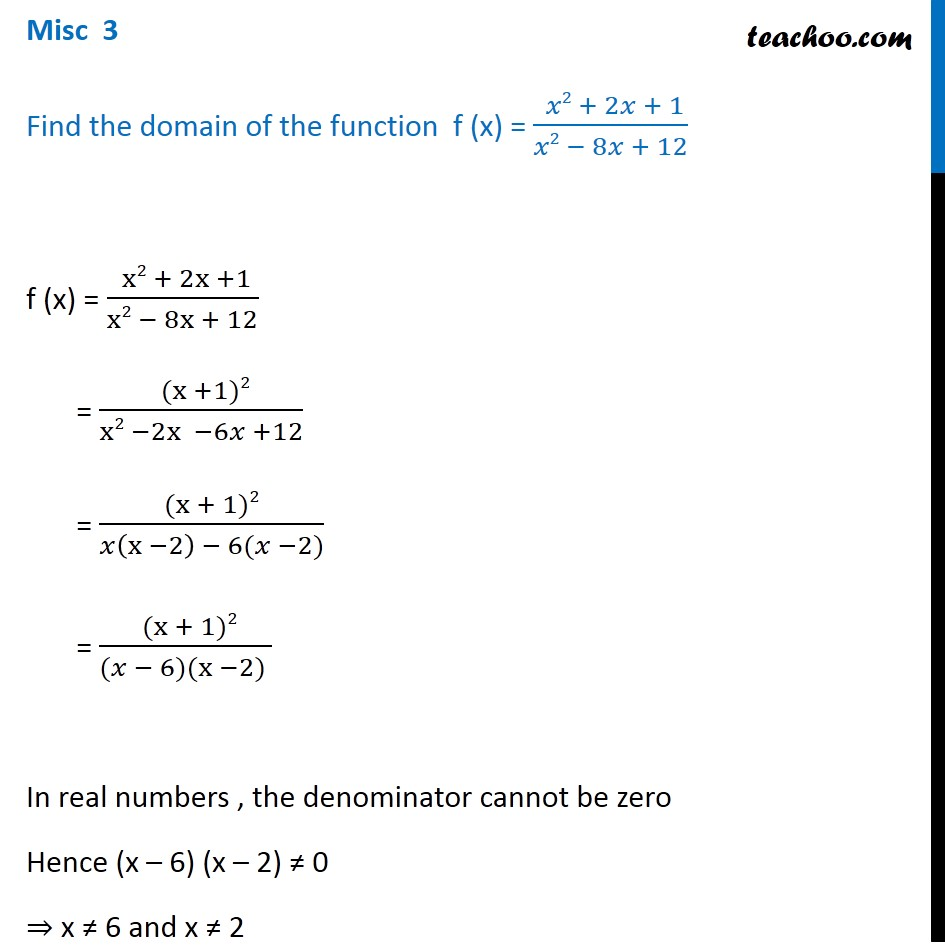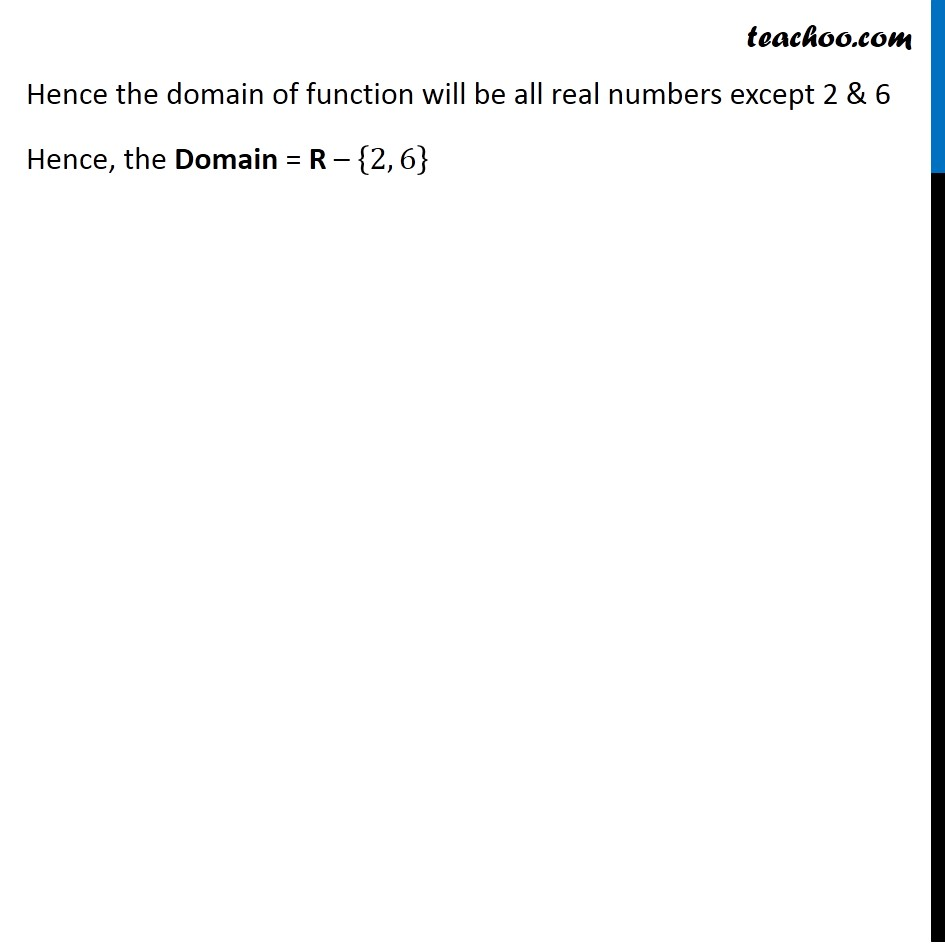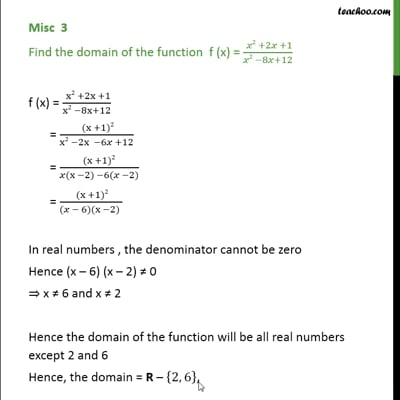Miscellaneous

Chapter 2 Class 11 Relations and Functions (Term 1)
Serial order wiseThis video is only available for Teachoo black users

### Transcript

Misc 3 Find the domain of the function "f" (x) = (" " 𝑥2 + 2𝑥 + 1)/(𝑥2 − 8𝑥 + 12) "f" (x) = (" " x2 + 2x +1)/(x2 − 8x + 12) = (" " (x +1)2)/(x2 −2x −6𝑥 +12) = ((x + 1)2)/(𝑥(x −2) − 6(𝑥 −2)) = (" " (x + 1)2)/((𝑥 − 6)(x −2) ) In real numbers , the denominator cannot be zero Hence (x – 6) (x – 2) ≠ 0 ⇒ x ≠ 6 and x ≠ 2 Hence the domain of function will be all real numbers except 2 & 6 Hence, the Domain = R – {2, 6}